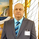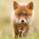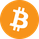7520 visitas
7520
EXPERIMENTAL:
fan projection from zigzag .
```study(title='[RS]PA Zigzag Fibonacci FAN V0', shorttitle='F', overlay=true)
useHA = input(false, title='Use Heikken Ashi Candles')
useAltTF = input(true, title='Use Alt Timeframe')
tf = input('D', title='Alt Timeframe')

zigzag() =>
_isUp = close >= close
_isDown = close <= close
_direction = _isUp and _isDown ? -1 : _isDown and _isUp ? 1 : nz(_direction)
_zigzag = _isUp and _isDown and _direction != -1 ? highest(2) : _isDown and _isUp and _direction != 1 ? lowest(2) : na

_ticker = useHA ? heikenashi(tickerid) : tickerid
sz = useAltTF ? (change(time(tf)) != 0 ? security(_ticker, tf, zigzag()) : na) : zigzag()

plot(sz, title='zigzag', color=black, linewidth=2)

//  ||---   Pattern Recognition:

x = valuewhen(sz, sz, 1)
z = valuewhen(sz, sz, 0)
n_x = valuewhen(sz, n, 1)
n_z = valuewhen(sz, n, 0)

price_range = z-x
bar_range = n_z-n_x
step = price_range/bar_range
projection = sz ? na : z + ((n-n_z)*step)
projection_n0236 = sz ? na : z + ((n-n_z)*step)*-0.236
projection_n0382 = sz ? na : z + ((n-n_z)*step)*-0.382
projection_n0500 = sz ? na : z + ((n-n_z)*step)*-0.500
projection_n0618 = sz ? na : z + ((n-n_z)*step)*-0.618
projection_n1000 = sz ? na : z + ((n-n_z)*step)*-1.000
projection_n1618 = sz ? na : z + ((n-n_z)*step)*-1.618
projection_n3141 = sz ? na : z + ((n-n_z)*step)*-3.141
plot(title='0.0', series=projection, style=linebr, color=blue)
plot(title='-0.236', series=projection_n0236, style=linebr, color=gray)
plot(title='-0.382', series=projection_n0382, style=linebr, color=yellow)
plot(title='-0.500', series=projection_n0500, style=linebr, color=orange)
plot(title='-0.618', series=projection_n0618, style=linebr, color=red)
plot(title='-1.000', series=projection_n1000, style=linebr, color=black)
plot(title='-1.618', series=projection_n1618, style=linebr, color=maroon)
plot(title='-3.141', series=projection_n3141, style=linebr, color=aqua)
```Hi Ricardo:

Where the variable n comes from in: n_z = valuewhen(sz, n, 0) as I dont see it been declared.
Thanks.
Responder@RicardoSantos

Request:

Ability to only show the most recent fan. For example, if I set the time frame of the indicator to 1 hour, it would be great it only the current fan that is currently being drawn is shown. Having the option to choose this in the settings would be really great, if possible, and if you are willing! Thanks.
Responderstockspy
@stockspy, thats not possible to do, its a restriction on how pinescript works.
ResponderThis is quite amazing. If only wanting to use it as minimally as possible, it appears to be useful in directing you as to which overall direction the market is trending (the fan is fanning upwards in an uptrend, and fanning downwards in a downtrend). I've noticed days where the market could surge upwards during the day, but the overall fan stays pointed downwards, and the market ultimately lowers back down at least a bit from it's intraday high.

Although, I haven't witnessed this yet during live trading, since I just found this great scipt, so looking forward to that!
ResponderHi. RicardoSantos. Does it repaint?

thank you for making this masterpiece :)
Responder